Maths-
General
Easy

Question

# A dome of a building is in the form of a hemisphere. From inside it was white washed at the cost of RS 4989.60. If the cost of white washing is Rs 20 per square metre , find the i) inside surface area of the dome ii) Volume of the air inside the domeHint:

## The correct answer is: 179.67cm3

### Explanation: We have given a dome of a building is in the form of a hemisphere. From inside it was white washed at the cost of . If the cost of white washing is per square metre. We have to find the (i) Inside surface area of dome. (ii) Volume of the air inside the dome Step 1 of 1:It is given that the total cost of white washing is Rs 4989.60.The cost of white washing per unit square meter is Rs 20.So,Total inside surface area will be=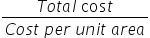=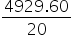= 246.48m2Step 2 of 2:Now inside surface area is 246.48m2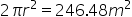r2 = 39.22r = 6.26So, The volume will be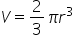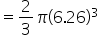= 82.07Hence, The inside surface area is 246.48m2 and the inside volume is 82.07m3#### With Turito Foundation.#### Get an Expert Advice From Turito.